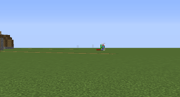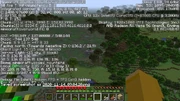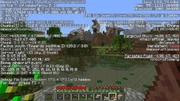# Tutorials/Measuring distance

Measuring distances in Minecraft can be quite tedious, but with a few simple guidelines you will never make a mistake again!

## Metric Units

Distances in Minecraft are quite easy to measure. Officially , Minecraft uses the metric system, and each block is considered to be 1 cubic metre. When you measure long distances, it's easier to count if you mark the terrain with a space of 4 blocks between each marked block. The first marker represents a zero. Every second marker (ignoring the zero-mark) is then a 10-meter mark. Make a distinguished mark at the 21st marker to represent a hundred (100), because the first marker block represents zero (0). Use a sign to mark larger numbers to save time and resources.

(ZERO) 1 2 3 4 (MARK) 1 2 3 4 (10 METERS) 1 2 3 4 (MARK) 1 2 3 4 (20 METERS) ...

## Imperial/U.S. Customary Units

Assume that one block's length is 1 yard. 1 mile is exactly 1760 yards. To measure 176 yards (1/10 of a mile), place your zero mark, then leave 7 block space between your next marker. Every second marker (ignoring the zero-mark), is 16 yards. Make a distinguished mark at the 11th 16 yards mark (zero-mark not included),

(ZERO) 3 + 1 + 3 (MARK) 3 + 1 + 3 (16 YARDS) 3 + 1 + 3 (MARK) 3 + 1 + 3 (32 YARDS) ... (176 YARDS) 3 + 1 + 3 (MARK) 3 + 1 + 3 (16 YARDS) ...

-------------------------------------------------- 1 ----------------------------------------------- 2 ----- ... ----- 11 ---------------------------------------------- 1 ----- ...

---------------------------------------------------------------------------------------------------------------------- 1/10 mile ---------------------------------------------------- ...

If you want to ignore the yard unit:

One mile converts to 1609.344m. Consider a Mile to be 1610 blocks long, for the sake of Minecraft practicality. Use the metric method (4 block spacing between). Make 161 x 10th meter marks. Use a sign to mark larger numbers to save time and resources.

In Real Life, 1 Mile = 1.6 km & 0.625 Mile = 1 km.

## GPS

If you press F3, the debug screen will give you your present location in X, Y and Z coordinates. Measuring distances between two locations or waypoints is as easy as subtraction, if you walk in a cardinal direction. Otherwise you will need to make use of the Pythagorean theorem to compute the distance. This is not strictly in-game, but it makes a drastic difference in gameplay, avoiding a lot of frustrated wandering. Note that the X and Z coordinates are horizontal and can be positive or negative (the spawn point will be fairly close to 0, 0), but the Y coordinate represents your altitude, and Y=0 is the bedrock floor of the gameworld.

## Relative to Green LineMeasuring in the debug screen(magnified). The human hitbox is as high as the green line, meaning he stands about 62 blocks away from the player.

This method is much faster and requires no building, though may not be 100% accurate. After going into the debug screen, the crosshairs are replaced with 3 colored lines. Take the length of the green line(which is always the same if you face near 0 on the Y-Axis) relative to a block. If one normal sized block is the same height as the green line, the player is standing about 35 blocks away from it. If it's 1.5 blocks long 52-53 blocks away, 2 blocks long 70 blocks away etc.
Humans and mobs can also be used for measurement. Pressing F3 + B will show hitboxes. If the green line is as high as a player hitbox, he is about 62 blocks away. With this, observable distances can be measured very quickly. However, aiming upwards or downwards will shrink the green line and that has to be accounted for if one measures distances far above or below him.

The crosshair or the blue and red line can of course also be used, but as the crosshair is slightly transparent and the blue/red line changes with the X-Axis, they are obviously not recommended unless you're playing before 1.8 or are trying to measure distances at a 90° angle above or below you, respectively.

Note that the green line changes size relative to the GUI, and was a different size entirely in 1.8. These numbers assume a normal sized GUI.

## Calculating coordinates of distant locationsFinding the X and Z coordinates of a distant Amethyst Geode, noting the player's position of "XYZ: -743.349 / 137.0000 / 540.144" and and the target's direction and tilt angle (-136.2 / 21.9)

Calculate a distant object's X and Z coordinates using the tilt angle in the debug screen by estimating the target's elevation from a vantage point significantly higher or lower in elevation. Typically you would pillar up about 50 to 60 blocks, or climb a tall mountain, and use the direction and downward tilt angle of the target with your coordinates to calculate first the horizontal distance to the target, then the X and Z coordinates of the target based on that distance and direction.

Pointing at the target, using FOV 30 or a spyglass for greatest accuracy, and the debug screen on, divide the difference in elevation by the tangent of the tilt angle. If you are on a high mountaintop at Y=137 and you see an Amethyst Geode in a swamp, you can estimate it is at sea level, or Y=63. The debug screen says you are at "XYZ: -743.349 / 137.0000 / 540.144" and two lines below that, you are looking at a target "Facing: north (Towards negative Z) (-136.2 / 21.9)". Your tilt angle is 21.9° and your rotation is 360 - 136.2 = 223.8°, converting from Minecraft's -180°/+180° notation to conventional 0° to 360° notation. The elevation difference, ∆Y, is 137 - 63 = 74. Find the distance by dividing ∆Y by the tangent of the tilt angle: 74 ÷ tan (21.9) = 74 ÷ 0.402 = 184.08 blocks.

Your vector to the geode is 184 blocks facing the direction -136.2, or 223.8°. To find the coordinate components of this vector, multiply the distance times the sine of the direction for the ∆X, adding that to your X position, and multiply the distance times the cosine of the direction for the ∆Z, adding that to your Z position. The geode's ∆X is 184.08 ∙ sin (223.8) = 184.08 ∙ -0.692 = -127.41. Subtract this from your X, so -743.349 - (-127.41) = 615.93. The ∆Z is 184.08 ∙ cos (223.8) = 184.08 ∙ -0.723 = -132.86. Add this to your Z, 540.144 + (-132.86) = 540.144 - 132.86 = 407.28.Standing on the geode, the target's real coordinates were -618 67 408. The elevation is four blocks higher than sea level, and with this correction the calculation comes a couple blocks closer.

If you're confused whether to add or subtract for your X and Z values, use the debug screen for a sanity check, and note again "Facing: north (Towards negative Z)". The target's Z is somewhat less than your Z value of 540, hinting that you want to subtract 132 from 540 to get 407. If you turn a little to the right, you can verify the target is east of you, "towards positive X", so you're looking for a number greater than (i.e. less negative than) your X position of -743, so you want to subtract negative 127 from -723, which is -734 + 127 = -615.

Keep in mind that small errors can be magnified at great distances. Each one block error in your estimate of the target block's elevation can cause errors of several blocks in the X and Y coordinates, though generally for things you can see within 20 to 30 chunks, it's close enough that you can see the target when you get there.

## Conserving Markers

If the measurement is being taken above ground, and lighting the entire path is not necessary, place the markers as above. When 100 m is reached, the 10 m markers can be removed and reused for the next 100 m run. This allows for the path to be constructed without having to count 100 blocks at a time, while still allowing the markers on the completed path to be easily followed without using too much material.

## Volume and Surface Area

The formula for the volume of a cube is s3, where s stands for the measurement of one of the cube's side. Since each side of a normal Minecraft block is 1 metre, this would equal 13, which would result in 1m3. (This works the same for yards, or any other unit of length. So do the rest of these comments.)

The formula for the sa of a cube is 6s2, where s stands for the measurement of one of the cube's side. Since each side of a normal individual Minecraft block is 1 meter, this would equal 6×12, which would equal 6×1, which would result in 6m2.

As you make something, e.g. a house, bigger in all directions, its surface area increases faster than its length, but not as fast as its volume. The surface area tells you how many blocks you'll need for the outer walls, but your interior furnishings will probably increase according to the volume. Of course, shape matters: An 8×8×1 layer of dirt corresponds to a stack of 64 dirt blocks, but so does a 4×4×4 cube, or a 2×2×16 trench or shaft.

## Using the Euclidean distance formula

Sometimes the need arises in which you need to measure distances that don't align with the X or Z axes, which is easy to do with a little algebra. The formula for Euclidean distance (in two dimensions), where d is the distance:Where:

• d = Distance in meters
• x1, z1 = Location number 1, in meters
• x2, z2 = Location number 2, in meters

### Example

Suppose the F3 debug screen shows the following at Location 1:

XYZ: -35.313 / 68.00000 / 97.489


These numbers are coordinates in meters. At Location 2, it shows:

XYZ: 76.793 / 43.00000 / -5.113


Usually, the decimal points can be truncated (ignored), as usually you don't want to cloud your results with where you happen to be standing within each block. In two-dimensional (map) coordinates, we also ignore the elevation (Y value). Hence, those two screens give us the following coordinates:

x1, z1 = -35, 97
x2, z2 =  76, -5


Now we simply plug those numbers in to the distance formula, above:Considering horizontal (map) distance only, the two locations are 150.75 m apart.

### Euclidean distance in 3 dimensions (including elevation)

The above calculation is correct if you want the "map" distance between two points (i.e., only the north–south (z) and East/West (x) distance). But if you wish to include the elevation (y) in the distance calculation as well, that's very easy to do: Simply add the y coordinates to the above distance formula:Referencing the above debug screens again, our 3-dimensional coordinates are as follows:

x1, y1, z1 = -35, 68, 97
x2, y2, z2 =  76, 43, -5


Again, solving for d:With the elevation considered, the two locations are 152.8 m apart. Note that, in this example, including the 25 m elevation resulted in a difference of about 2 m (2 blocks).